﻿ Semiconductor, GaAs by kp Kane method

# Semiconductor by quantum mechanics

## Unreal spin is Nobel prize candidate ?

### [ If Nobel prize is given to fictional "spin", it destroys science ! ]

(Fig.1)  Spin-orbit interaction really happens in semiconductor ?Electron spin in solids (= spintronics ? ) often becomes Nobel prize candidate as a form of "spin-Hall" and "topological insulator".

But electron spin is physically impossible. A tiny point-like electron must rotate at far faster-than-light speed to generate its angular momentum !

Then why does Nobel committee try to give their prestigious prize to unrealistic spin ?  In other words, without Nobel prize, spintronics is useless.

## Quantum mechanics stops science !

### [ Experimental Data → fictional quasiparticle, effective mass ! ]

(Fig.2)  We must use experimental data to predict "real concept" !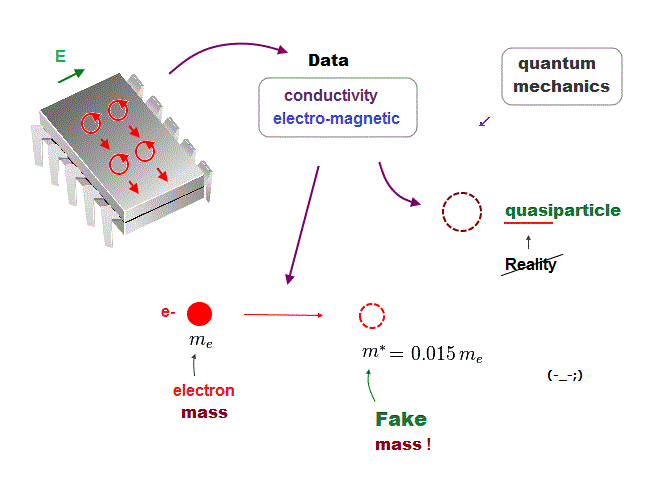In the present quantum mechanics, even when we get some new experimental data, we cannot use them to clarify real mechanisms !

Instead, quantum mechanics forces us to use these data to create artificial quasiparticle, effective mass ..  So our science stops its progress.

This is a very serious problem.  We must address it immediately not to increase more educational victims under false science.

## Schrodinger equation cannot handle multi-electrons.

### [ They just choose trial wavefunction as "fake" solutions. ]

(Fig.3)  Choosing convenient wavefunction from infinte choices is nonsense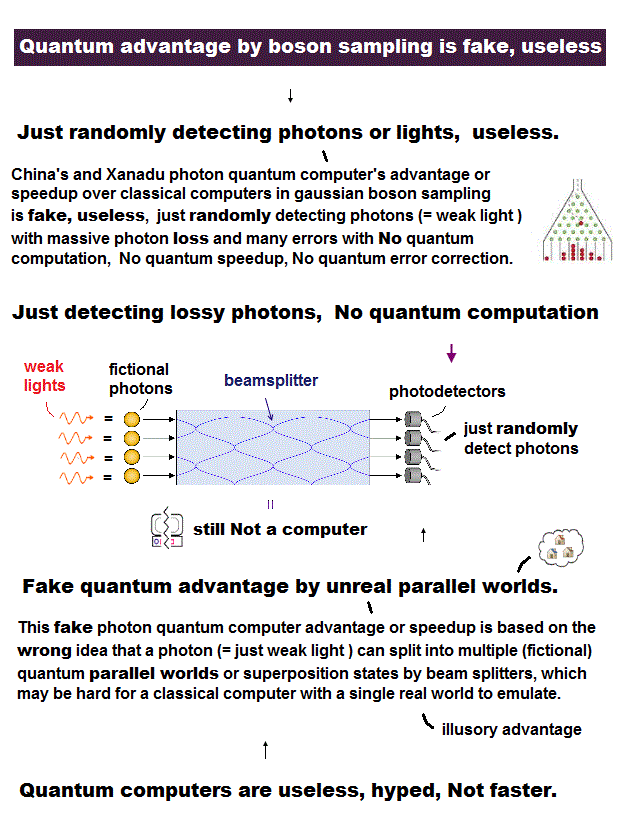Schrodinger equation cannot be solved in multi-electron atoms except for one-electron hydrogen atom.

So all physicists can do is choose approximate trial function.  The problem is there is NO restriction in choosing this trial function as candidate.

We can choose convenient form of trial wavefunction out of infinite choices.  So it's impossible to compare infinite possible trial functions giving the lowest energy.

This is the reason why Schrodinger equation is useless, has NO power to predict physical values forever, also in DFT and Nobel prize.

## Quantum mechanics relies on "fake" mass, spin !

### [ "Effective" mass, spin-orbit are "unreal" concepts. ]

(Fig.4)  ↓ Fake mass, fake spin g-factor, fake spin-orbit = semiconductor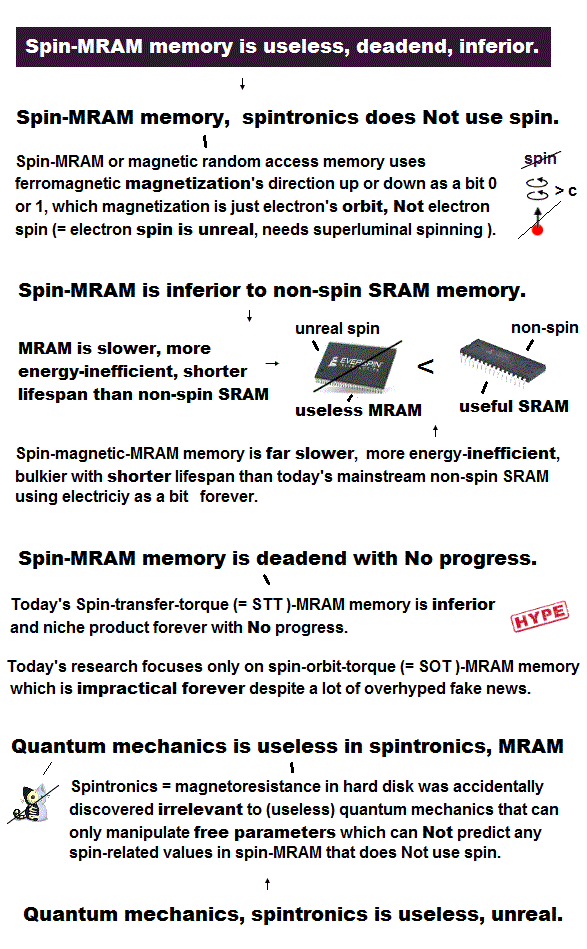Schrodinger equation in quantum mechanics cannot solve multi-electrons, so they just choose fake trial wavefunction.  It means useless.

In solid-physics, they adopt band theory where they replace complicated actual solid electrons by a free single particle with a unreal mass ( this p.13 ).

Of course, this band theory is a false approximation.  It relies on unreal effective mass, which can be smaller than the actual electron mass or negative ( this p.5 )

Spin g-factor and spin-orbit coupling are all unreal (= called "effective", see this p.8 ) in semiconductor by quantum mechanics.

## Unreal (= effective ) mass in semiconductor

### [ Effective mass smaller than a real electron's mass (= m0 ) ? ]

(Fig.5)  Effective mass in GaAs = 0.0665 m0 (= electron mass ) !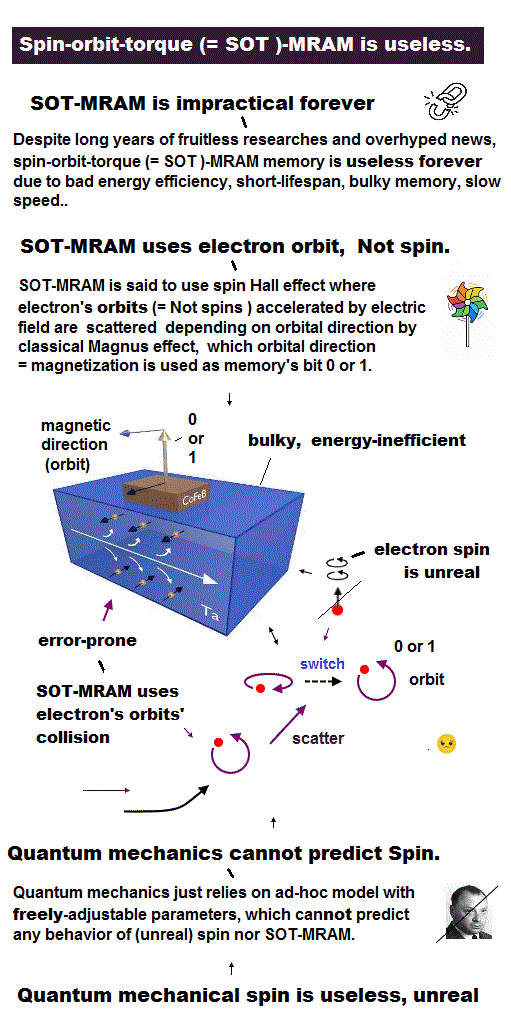Gallium arsenide (= GaAs ) is a compound semiconductor, which is used as research topic more frequently than any other semiconductors.

Quantum mechanics cannot handle multi-electron atoms and solids. So all physicistis can do is adopt a fake free particle with unreal mass and spin g-factor !

This "fake mass" is called "effective mass (= m* )", which can be smaller and larger than the actual electron mass (= m0 ).

For example, effective mass inside GaAs is smaller (= 0.066 m0 this p.13 ) than the actual electron mass.

The actual electron spin g factor must be "2", but effective g-factor is less than that ( g* = -0.44, see this p.43 ).

So quantum mechanics depends on unreal concepts, which means "useless" !

## Spin-orbit interaction is relativistic effect ?

### [ Moving spin in "electric" field feels "magnetic" field ? ]

(Fig.6)  Electric → magnetic in rest frame of moving electron spin ?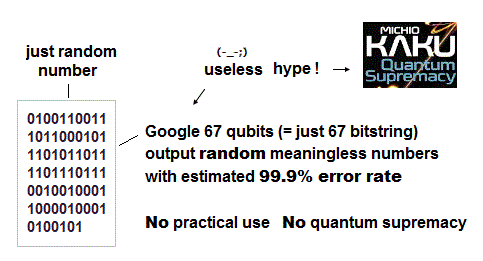Spin-orbit interaction is thought to be one of Einstein relativistic effect ( this p.7 ), though faster-than-light spin itself violates relativity.

Einstein said when an electron (= spin S ) is moving in the electric field (= ∇V), it feels the magnetic field (= B ) in its rest frame.

Using Lorentz transformation of electromagnetic fields, we can get the strength of spin-orbit coupling (= λ = -3.7 × 10-6 ), which is very week.

## Quantum mechanics uses "fake" spin-orbit !

### [ Quantum semiconductor adopts unreal spin-orbit coupling. ]

(Fig.7)  Effective spin-orbit coupling is far bigger than Einstein relativity !Though they claim spin-orbit interaction proves Einstein relativistic effect, physicsits adopts fake spin-orbit effect completely different from relativity !

They often use "kp approximation" in semiconductors which relies on unreal effective spin-orbit equation ( this p.8, this p.3 ).

In this fake theory, spin-orbit interaction in GaAs is by six orders of magitude stronger than in Einstein theory and has the opposite sign ( this p.6, this p.2 )

So when you see "spin-orbit in solids" in various websites, it means neither actual spin nor Einstein relativity !

These false parameters are artificially adjusted to fit experimets, so useless ( this p.2 ).

## Quantum mechanics is stalled in fake concepts.

### [ Semiconductors rely on unreal effective mass and quasiparticle ! ]

(Fig.8)  Effective mass, g-factor, spin-orbit are unreal.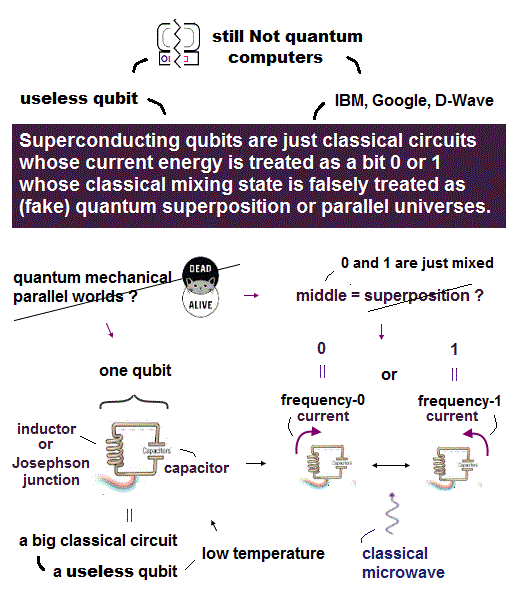Schrodinger equation in quantum mechanics cannot handle multi-electron atoms and molecules, because this theory was invented in ancient times (= 1920s ).

So for useless quantum mechanics to treat solids and semiconductors, it has to rely on unreal concepts such as effective mass, spin-orbit and quasiparticle.

As long as Nobel prize keeps awarded to unreal concepts, this prize keeps contributing to destroying young strudents' career as a convenient tool of scientific fraud !2016/7/1 updated. Feel free to link to this site.